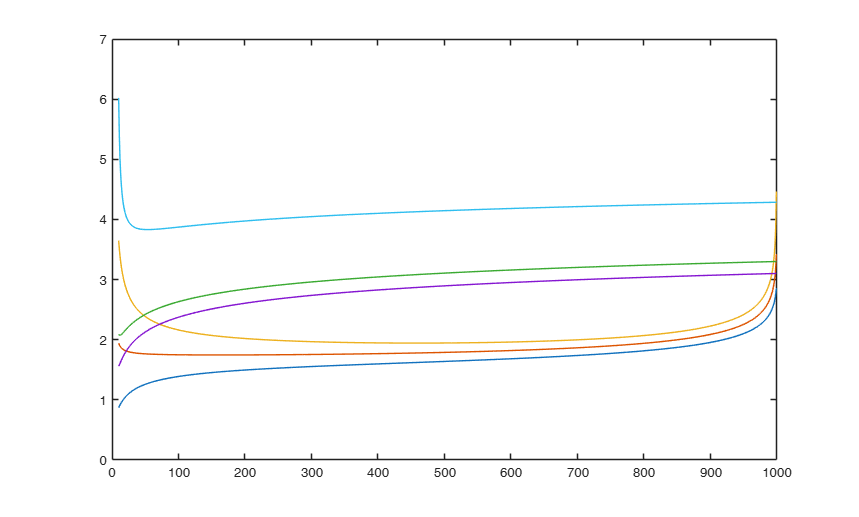FSRbonfbound

FSRbonfbound computes Bonferroni bounds for each step of the search (in linear regression)

Syntax

• Bbound=FSRbonfbound(n,p)example
• Bbound=FSRbonfbound(n,p,Name,Value)example

Description

Bbound =FSRbonfbound(n, p) mdr with Bonferroni envelopes.

Bbound =FSRbonfbound(n, p, Name, Value) mdr with personalized Bonferroni envelopes.

Examples

expand allmdr with Bonferroni envelopes.

Example of creation of 1, 50 and 99 per cent envelopes based on 1000 observations and 5 explanatory variables using exact method

MDRenv = FSRenvmdr(1000,5,'init',10);
Bbound = FSRbonfbound(1000,5,'init',10);
plot(MDRenv(:,1),MDRenv(:,2:end),Bbound(:,1),Bbound(:,2:end));mdr with personalized Bonferroni envelopes.

Example of creation of 1, 50, 99 and 99.9 per cent envelopes based on 1000 observations and 5 explanatory variables using exact method

MDRenv = FSRenvmdr(1000,5,'init',10,'prob',[0.01 0.5 0.99 0.999]);
Bbound = FSRbonfbound(1000,5,'init',10,'prob',[0.01 0.5 0.99 0.999]);
plot(MDRenv(:,1),MDRenv(:,2:5),Bbound(:,1),Bbound(:,2:5));

Input Arguments

n — number of observations. Scalar.

Number of observations on which the envelopes are based.

Data Types: single| double

p — number of explanatory variables (including the intercept if present). Scalar.

Number of expl. variables on which the envelopes are based.

Data Types: single| double

Name-Value Pair Arguments

Specify optional comma-separated pairs of Name,Value arguments. Name is the argument name and Value is the corresponding value. Name must appear inside single quotes (' '). You can specify several name and value pair arguments in any order as Name1,Value1,...,NameN,ValueN.

Example: 'init',100 starts monitoring from step m=100 , 'prob',[0.01 0.99]

init —Search initialization.scalar.

It specifies the point where to initialize the search and start monitoring minimum deletion residual. if init is not specified it will be set equal to :

p+1, if the sample size is smaller than 40;

min(3*p+1,floor(0.5*(n+p+1))), otherwise.

Example: 'init',100 starts monitoring from step m=100

Data Types: double

prob —quantiles for which envelopes have to be computed.vector.

1 x k vector containing quantiles for which envelopes have to be computed. The default is to produce 1%, 50% and 99% envelopes.

Example: 'prob',[0.01 0.99]

Data Types: double

Output Arguments

Bbound —Bonferroni forward envelopes of mdr. Matrix

Matrix with n-m0+1 rows and length(prob)+1 columns:

1st col = fwd search index from m0 to n-1, 2nd col = bound for quantile prob, 3rd col = bound for quantile prob, ..., (k+1) col = bound for quantile prob[k].

Atkinson, A.C. and Riani, M. (2006), Distribution theory and simulations for tests of outliers in regression, "Journal of Computational and Graphical Statistics", Vol. 15, pp. 460-476.

Riani, M. and Atkinson, A.C. (2007), Fast calibrations of the forward search for testing multiple outliers in regression, "Advances in Data Analysis and Classification", Vol. 1, pp. 123-141.